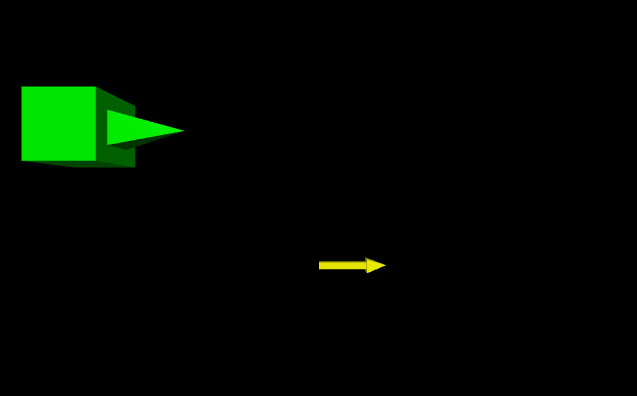# Making an arrow with VPython

`VPython` makes it easy to create navigable 3D displays and animations, even for those with limited programming experience. Because it is based on Python, it also has much to offer for experienced programmers and researchers. `VPython` allows users to create objects such as spheres and cones in 3D space and displays these objects in a window. This makes it easy to create simple visualizations, allowing programmers to focus more on the computational aspect of their programs. The simplicity of `VPython` has made it a tool for the illustration of simple physics, especially in the educational environment.

Installation :

`pip install vpython`

A arrow is a geometrical object in three-dimensional space that is a straight line(or cuboid) with a pointed end. We can generate an arrow in `VPython` using the `arrow()` method.

## arrow()

Syntax : arrow(parameters)

Parameters :

• pos : It is the position of the beginning of the shaft of the arrow. Assign a vector containing 3 values, example pos = vector(0, 0, 0)
• axis : It is the axis of alignment of the arrow. Assign a vector containing 3 values, example axis = vector(1, 2, 1)
• up : It is the orientation of the arrow. Assign a vector containing 3 values, example up = vector(0, 1, 0)
• color : It is the color of the arrow. Assign a vector containing 3 values, example color = vector(1, 1, 1) will give the color white
• opacity : It is the opacity of the arrow. Assign a floating value in which 1 is the most opaque and 0 the least opaque, example opacity = 0.5
• shininess : It is the shininess of the arrow. Assign a floating value in which 1 is the most shiny and 0 the least shiny, example shininess = 0.6
• emissive : It is the emissivity of the arrow. Assign a boolean value in which True is emissive and False is not emissive, example emissivity = False
• texture : It is the texture of the arrow. Assign the required texture from the textures class, example texture = textures.stucco
• length : It is the length of the arrow. Assign a floating value, the default length is 1, example length = 10
• shaftwidth : It is the width of the shaft of the arrow. Assign a floating value, the default shaft width is 0.1*(length of arrow), example shaftwidth = 10
• headwidth : It is the width of the head of the arrow. Assign a floating value, the default head width is 2*shaftwidth, example headwidth = 10
• headlength : It is the length of the head of the arrow. Assign a floating value, the default head length is 3*shaftwidth, example headlength = 10

All the parameters are optional.

Example 1 :An arrow with no parameters, all the parameters will have the default value.

 `# import the module ` `from` `vpython ``import` `*` `arrow() `

Output :Example 2 :An arrow using the parameters color, opacity, shininess and emissivity.

 `# import the module ` `from` `vpython ``import` `*` `arrow(color ``=` `vector(``0``, ``1``, ``0``),  ` `      ``opacity ``=` `0.5``,  ` `      ``shininess ``=` `1``,  ` `      ``emissive ``=` `False``) `

Output :Example 3 :Displaying 2 arrows to visualize the attributes pos, shaftwidth, headwidth and headlength.

 `# import the module ` `from` `vpython ``import` `*` ` `  `# the first arrow ` `arrow(pos ``=` `vector(``-``3``, ``1``, ``0``), ` `      ``shaftwidth ``=` `1``, ` `      ``headwidth ``=` `1``, ` `      ``headlength ``=` `2``, ` `      ``color ``=` `vector(``0``, ``1``, ``0``)) ` ` `  `# the second arrow ` `arrow(pos ``=` `vector(``0``, ``-``1``, ``0``), ` `      ``color ``=` `vector(``1``, ``1``, ``0``)) `

Output :Example 4 :An arrow using the parameters axis and up.

 `# import the module ` `from` `vpython ``import` `*` `arrow(pos ``=` `vector(``-``2``, ``0``, ``0``), ` `      ``color ``=` `vector(``1``, ``0``, ``1``), ` `      ``axis ``=` `vector(``1``, ``2``, ``2``), ` `      ``up ``=` `vector(``-``1``, ``5``, ``2``)) `

Output :My Personal Notes arrow_drop_upCheck out this Author's contributed articles.

If you like GeeksforGeeks and would like to contribute, you can also write an article using contribute.geeksforgeeks.org or mail your article to contribute@geeksforgeeks.org. See your article appearing on the GeeksforGeeks main page and help other Geeks.

Please Improve this article if you find anything incorrect by clicking on the "Improve Article" button below.

Article Tags :

Be the First to upvote.

Please write to us at contribute@geeksforgeeks.org to report any issue with the above content.